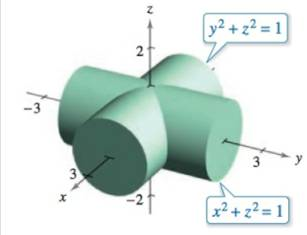Chapter 14.5, Problem 37E

Chapter
Section
Textbook Problem

Surface Area Find the surface area of the solid of intersection of the cylinders x 2 + z 2 = 1 and x 2 + z 2 = 1 (see figure).To determine

To calculate: The surface area of solid of intersection of area if the cylinders x2+z2=1 and y2+z2=1

Explanation

Given:

The given two cylinders are x2+z2=1 and y2+z2=1 as shown in the figure,

Formula used:

The surface area can be found by,

S=R1+[fx(x,y)]2+[fy(x,y)]2dA

The chain rule is:

ddxf(g(x))=f(g(x))g(x).

The differentiation formula is  ddx(xn)=nxn1, ddx(constant)=0.

Calculation:

The equation for the surface is,

z=1x2

First find the partial derivative with respect to x by the chain rule

ddxf(g(x))=f(g(x))g(x), ddx(xn)=nxn1, ddx(constant)=0.

The derivative is:

fx(x,y)=ddx(1x2)=(1x2)122(2x21)=x1x2

Againdifferentiate with respect to y is:

fx(x,y)=ddy(1x2)=0

Now put the above derivatives in the formula

Still sussing out bartleby?

Check out a sample textbook solution.

See a sample solution

The Solution to Your Study Problems

Bartleby provides explanations to thousands of textbook problems written by our experts, many with advanced degrees!

Get Started

In Exercises 4756, solve the given equation for the indicated variable. 423x=256

Finite Mathematics and Applied Calculus (MindTap Course List)

Evaluate: a. 2332 b. [(13)3]1/3

Applied Calculus for the Managerial, Life, and Social Sciences: A Brief Approach

In words, explain what is measured by SS, variance, and standard deviation.

Statistics for The Behavioral Sciences (MindTap Course List)

Solve the equation in Problem 1-6. 4.

Mathematical Applications for the Management, Life, and Social Sciences

In Exercises 110, evaluate the expression. P(52,7)

Finite Mathematics for the Managerial, Life, and Social Sciences

Given: m13=70 Find: m3

Elementary Geometry for College Students

0 1 π It does not exist.

Study Guide for Stewart's Multivariable Calculus, 8th

If 13f(x)dx=10 and 13g(x)dx=6, then 13(2f(x)3g(x))dx= a) 2 b) 4 c) 18 d) 38

Study Guide for Stewart's Single Variable Calculus: Early Transcendentals, 8th